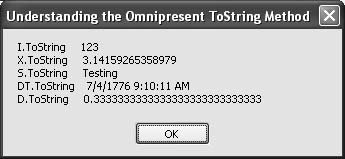# Recipe 5.36. Converting Any Data to a String

#### Problem

You have an instance of data and want to convert it to its default string representation.

#### Solution

Sample code folder: Chapter 05\UseToString

Use the ToString() method, which is included in all .NET objects, to return a general string for an object instance. To get you started, the following code demonstrates the default ToString() method on several types of variables:

` Dim someInt As Integer = 123 Dim someDouble As Double = Math.PI Dim someString As String = "Testing" Dim someDate As Date = #7/4/1776 9:10:11 AM# Dim someDecimal As Decimal = 1D / 3D Dim result As New System.Text.StringBuilder result.Append("someInt.ToString ") result.AppendLine(someInt.ToString()) result.Append("someDouble.ToString ") result.AppendLine(someDouble.ToString()) result.Append("someString.ToString ") result.AppendLine(someString.ToString()) result.Append("someDate.ToString ") result.AppendLine(someDate.ToString()) result.Append("someDecimal.ToString ") result.Append(someDecimal.ToString()) MsgBox(result.ToString()) `

#### Discussion

Figure 5-41 shows the results displayed by the sample code. Default formatting is used for all these ToString() methods.

The ToString() method is often overloaded to support a variety of formatting options, depending on the type of variable. This lets you convert doubles, for instance, to scientific or other formats. Check the Visual Studio online help resources for the ToString() method for each type of variable to discover the formatting options available.

All objects sport a ToString() method because all objects inherit it from System.Object. An example used repeatedly throughout this chapter is the StringBuilder class, which returns its internal character buffer converted to a string through its ToString() method.

##### Figure 5-41. Results of converting several variable types by using the ToString( ) method on eachAs you create your own classes, consider adding both a ToString() method and a corresponding Parse() method if the object's state can be represented as a string.Visual Basic 2005 Cookbook: Solutions for VB 2005 Programmers (Cookbooks (OReilly))
ISBN: 0596101775
EAN: 2147483647
Year: 2006
Pages: 400

Similar book on Amazon